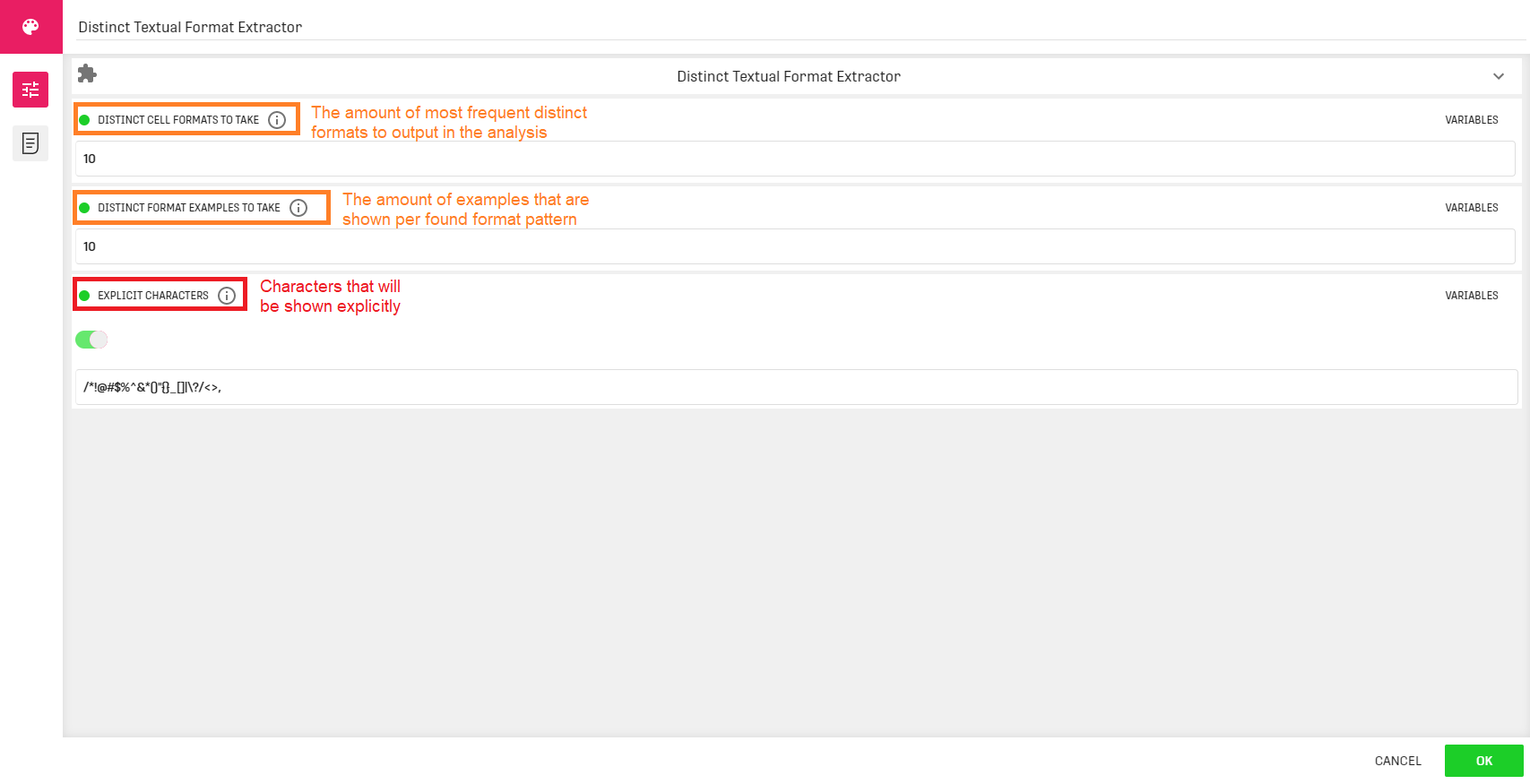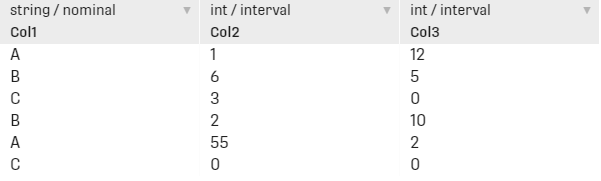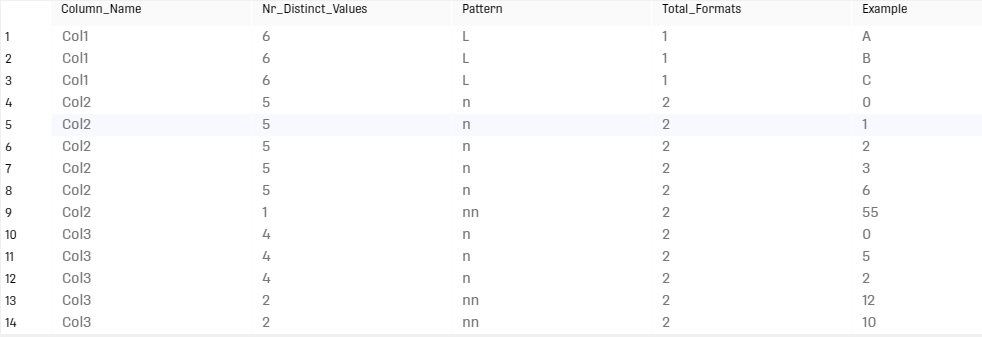# Overview

This processor creates summaries for every column in the input Dataset. Statistics include diverse patterns within a column along with the occurrence of each pattern, total number of formats and some example for each pattern.

# Configuration

This processor works with any given input dataset, and it can be configured as follows:# Output

The processor generates a table containing the following five columns:

• Column_Name: displays the column names form the input dataset
• Nr_Distinct_Values: number of occurrences of a specific pattern from a defined column
• Pattern: textual patterns within a defined column
• Total_Formats: number of different textual formats of a column
• Example: contains a value example for each of the patterns
NOTE THAT: the number of patterns to display will be determined by the first configuration field

# Example

In the following example the Distinct Textual Format Extractor processor will be used to extract textual description of a dataset inserted using a Custom Input Table.

For this example, we keep the default configuration.

## Example Input## ResultThe first column from the input dataset contains only uppercase alphabetical characters. As a result, there is only one textual format and one pattern 'L' with six occurrences.

The second column includes integers with two formats: 'n' meaning one digit and 'nn' meaning two digits. This column contains five signle digit numbers and only one double digit number (i.e: 55).

The third column is similar to the second one, except that it contains two double digit numbers and four single digit numbers.

# Related Articles

Distinct Textual Summary